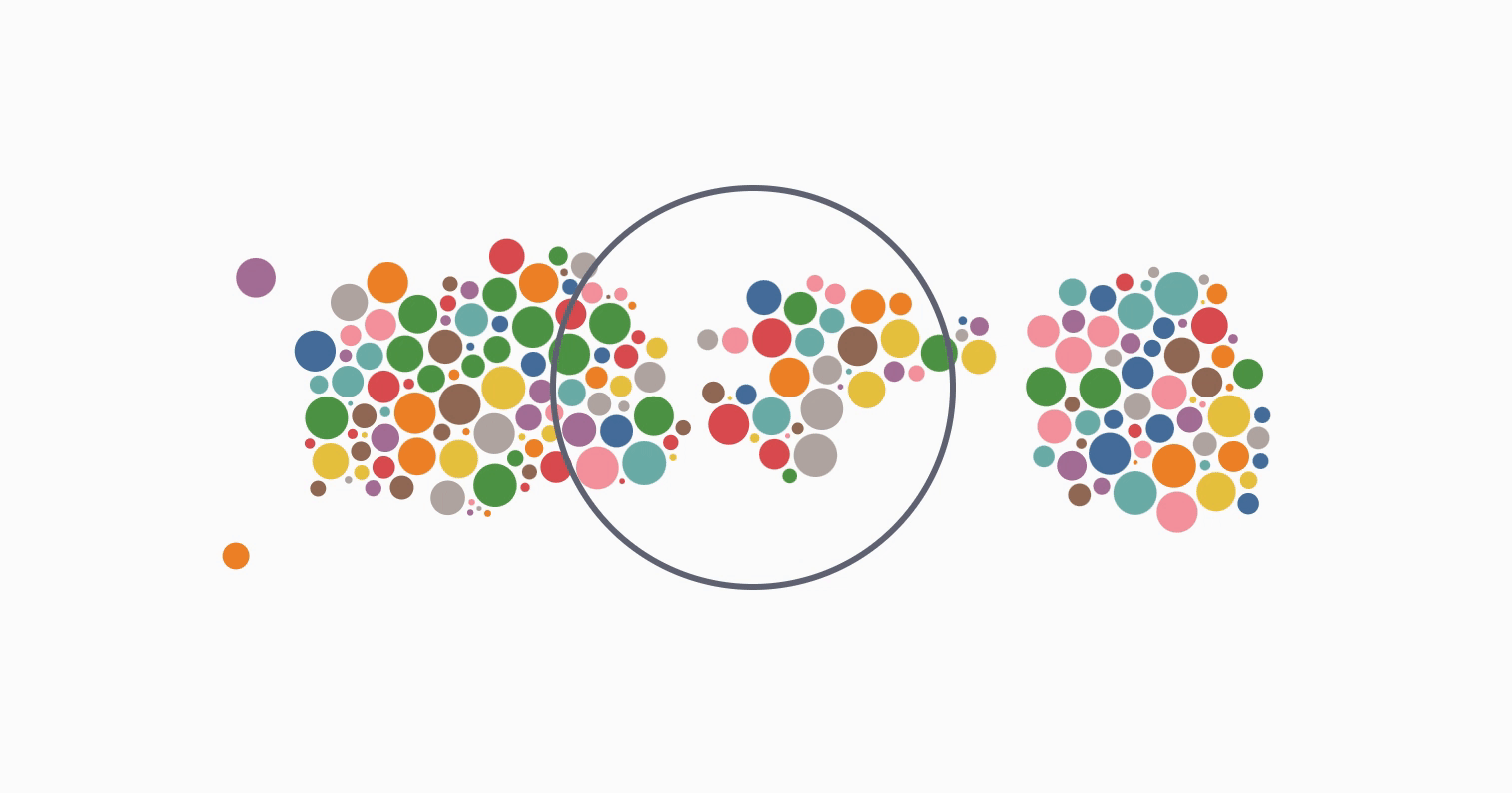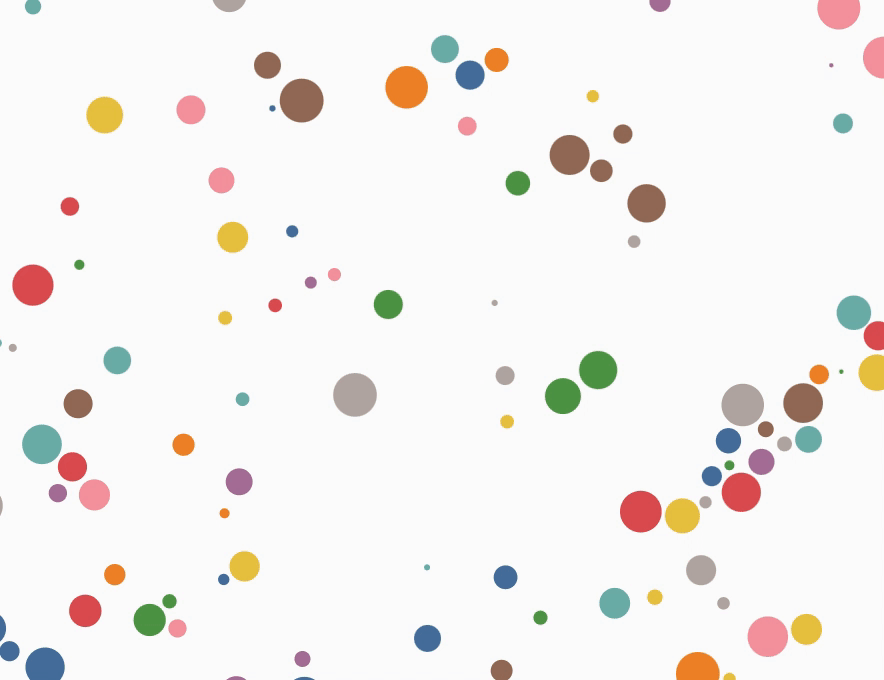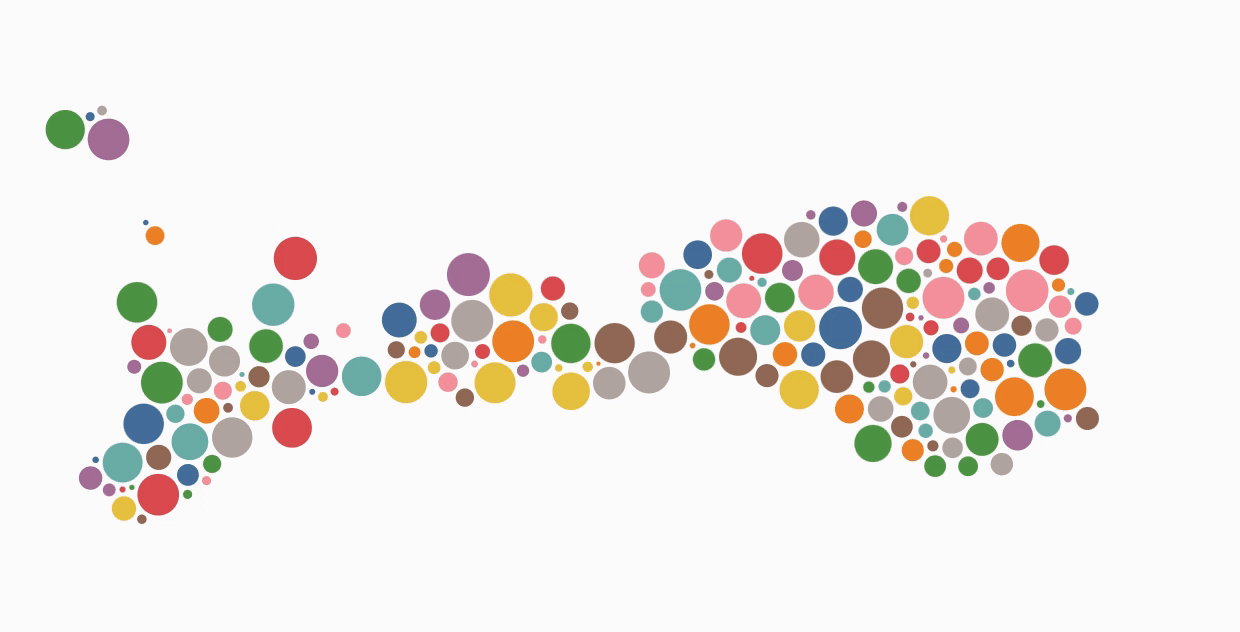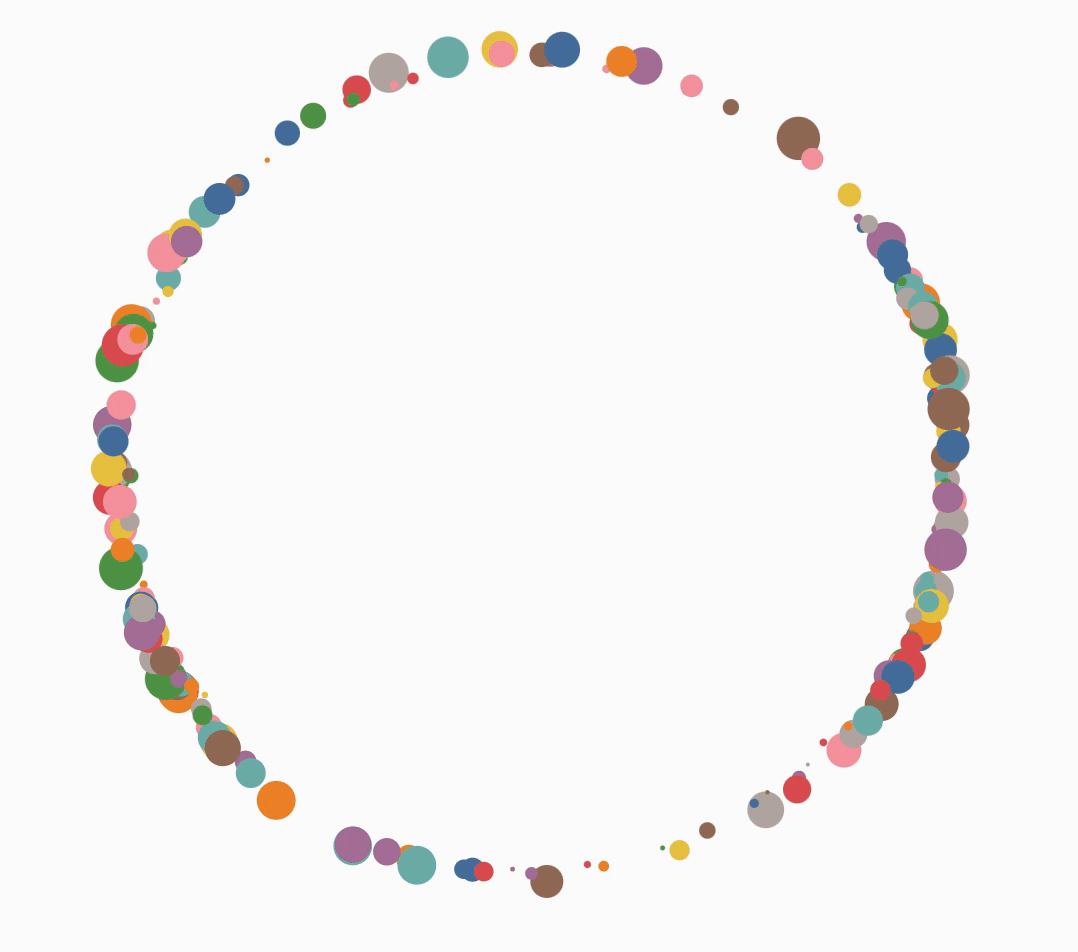# D3(v7)入门九：力向导图

## highlight: a11y-dark theme: vuepress## 力

D3 Force 是一种模拟物理运动原理的绘图算法，一开始给所有节点设置任意的初始值配置，接着根据配置的属性，让每个节点按属性去运动——这就是每个节点之间的力。

## 五种力

D3 一共给我们提供了五种力点击查看Demo:

### 向心力

• d3.forceCenter([xy]) – 创建一个中心作用力.
• center.x – 设置中心作用力的 x -坐标.
• center.y – 设置中心作用力的 y -坐标.### 碰撞力

• d3.forceCollide – 创建一个圆形区域的碰撞检测力模型.
• collide.strength – 设置碰撞检测力模型的强度.
• collide.iterations – 设置迭代次数.### 连接力(弹簧力)

• d3.forceLink – 创建一个 `link`(弹簧) 作用力.
• link.id – 设置边元素中节点的查找方式是索引还是 `id` 字符串.
• link.distance – 设置 `link` 的距离.
• link.strength – 设置 `link` 的强度.### 电荷力

• d3.forceManyBody – 创建一个电荷作用力模型.
• manyBody.strength – 设置电荷力模型的强度.
• manyBody.theta – 设置 `Barnes–Hut` 算法的精度.
• manyBody.distanceMin – 限制节点之间的最小距离.
• manyBody.distanceMax – 限制节点之间的最大距离.### 径向力

• radial.x – 设置环形作用力的目标中心 x -坐标.
• radial.y – 设置环形作用力的目标中心 y -坐标.## 力向导图

• d3.forceSimulation – 创建一个新的力学仿真.
• simulation.restart – 重新启动仿真的定时器.
• simulation.stop – 停止仿真的定时器.
• simulation.tick – 进行一步仿真模拟.
• simulation.nodes – 设置仿真的节点.
• 每个 node 必须是一个对象类型，下面的几个属性将会被仿真系统添加:
• `index` – 节点在 nodes 数组中的索引
• `x` – 节点当前的 x-坐标
• `y` – 节点当前的 y-坐标
• `vx` – 节点当前的 x-方向速度
• `vy` – 节点当前的 y-方向速度
• simulation.alpha – 设置当前的 `alpha` 值.
• simulation.alphaMin – 设置最小 `alpha` 阈值.
• simulation.alphaDecay – 设置 `alpha` 衰减率.
• 为0的话，永远都不会停
• simulation.alphaTarget – 设置目标 `alpha` 值.
• simulation.velocityDecay – 设置速度衰减率.
• simulation.force – 添加或移除一个力模型.
• simulation.find – 根据指定的位置找出最近的节点.
• simulation.on – 添加或移除事件监听器.
• `tick` – 仿真内部定时器每次 `tick` 之后。
• `end` – 当 alpha < alphaMin 时仿真内部定时器停止。
``````const simulation = d3.forceSimulation(nodes)
// 连接力
// 在 y轴方向上施加一个力
.force('y', d3.forceY().strength(0.025))
// 电荷力
.force('charge', d3.forceManyBody())
// 碰撞力
//  向心力
.force('center', d3.forceCenter(width / 2, height / 2))
``````

### 创建模拟数据

``````const nodes = [
{name: "张三"}
...
]

{ source: 0, target: 1, relation: "关系1"}
...
]
``````

### 创建力模型

``````let simulation = d3.forceSimulation(nodes)
``````

### 绘制节点和连线

``````// 画线
function drawLine() {
let lines = svg.append("g")
.selectAll(".force-line")
.enter()
.append("line")
.attr("class", "line")
.attr("stroke", "#999")
.attr("stroke-width", "1px");
return lines;
}
let lines = drawLine();

// 画节点节点盒子

function drawCircle() {
let nodeGroups = svg.append("g")
.attr("class", "nodes-box")
.selectAll(".force-node")
.data(nodes)
.enter()
.append("g")
.attr("class", "force-circle")

nodeGroups.append("circle")
.attr("class", "force-circle")
.attr("r", 20)
.style("fill",(d, i) => color(i));

nodeGroups.append("text")
.attr("class", "force-text")
.attr("dy", ".33em")
.attr("font-size", "12px")
.attr("text-anchor", "middle")
.style("fill", "#eee")
.text(d => d.name);
return nodeGroups;
}
let nodesCircle = drawCircle();
``````### 监听 tick

``````let simulation = d3.forceSimulation(nodes)
.on("tick", ticked);

function ticked()
lines
.attr("x1", (d) => d.source.x)
.attr("y1", (d) => d.source.y)
.attr("x2", (d) => d.target.x)
.attr("y2", (d) => d.target.y);

// 这里就不适合 去改变circle的圆心位置了，因为有文字存在，改变整个circleGroup的transform
nodesCircle.attr("transform", function (d) {
// d.fx=d.x;d.fy=d.y; 固定位置
return "translate(" + d.x + ", " + d.y + ")";
});
}
``````### 添加向心力

``````let simulation = d3.forceSimulation(nodes)
.force("center", d3.forceCenter(width / 2, height / 2)) // 用指定的x坐标和y坐标创建一个居中力
.on("tick", ticked);
``````### 添加碰撞力

``````let simulation = d3.forceSimulation(nodes)
.force("charge", d3.forceManyBody().strength(-200)) // 电荷力 相互之间的作用力
.force("center", d3.forceCenter(width / 2, height / 2)) // 用指定的x坐标和y坐标创建一个居中力
.on("tick", ticked);
``````### 添加拖拽效果

`d3.drag`后面再详细介绍，本章就不深入了。

``````let nodeGroups = svg.append("g")
...
.call(
d3.drag().on("start", started).on("drag", dragged).on("end", ended)
);
// 拖拽
function started(event) {
if (!event.active) simulation.alphaTarget(0.3).restart();
event.subject.fx = event.subject.x;
event.subject.fy = event.subject.y;
}

function dragged(event) {
event.subject.fx = event.x;
event.subject.fy = event.y;
}

function ended(event) {
if (!event.active) simulation.alphaTarget(0);
event.subject.fx = null;
event.subject.fy = null;
}
``````1. 连接力：拖拽任意节点，其余节点都会同向运动
2. 碰撞力：在拖拽的过程中，各节点之间不会重合
3. 向心力：拖拽不能拖到任意位置，由于向心力的存在，最后还是会向中心靠拢

## 总结